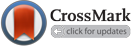SALAR, A., Jassim, A. (2022). Use Different Mathematical Methods to Solve Three Dimensional Conduction Heat Equation in Cartesian Coordinate. , 16(2), 11-14. doi: 10.33899/csmj.2022.176584
AHMED SALAR; Ahmed M.J. Jassim. "Use Different Mathematical Methods to Solve Three Dimensional Conduction Heat Equation in Cartesian Coordinate". , 16, 2, 2022, 11-14. doi: 10.33899/csmj.2022.176584
SALAR, A., Jassim, A. (2022). 'Use Different Mathematical Methods to Solve Three Dimensional Conduction Heat Equation in Cartesian Coordinate', , 16(2), pp. 11-14. doi: 10.33899/csmj.2022.176584
SALAR, A., Jassim, A. Use Different Mathematical Methods to Solve Three Dimensional Conduction Heat Equation in Cartesian Coordinate. , 2022; 16(2): 11-14. doi: 10.33899/csmj.2022.176584